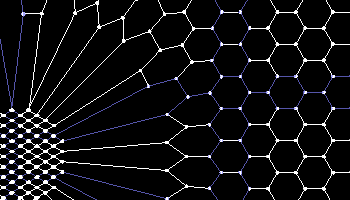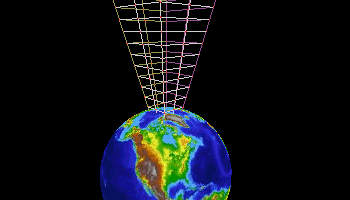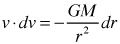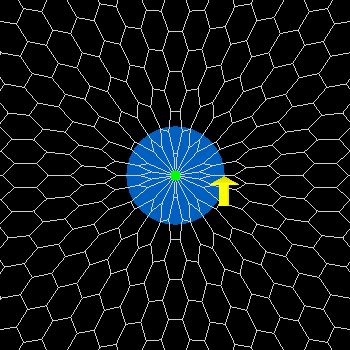"There is no theory of gravity beyond the mathematical form."

- Richard Feynman -

## Gravity

You may have noticed when viewing the particle forming simulation, that as soon as the nodes collapsed to form a particle, the ether immediately started to rush inward. This inward flow of ether is what causes gravity. To be more precise, the 'accelerating inward flow' of ether is gravity. I have created two more simulations that illustrate this a little more clearly: one shows ether rushing in from a distance (Figure 8a) and the other is a close-up of ether rushing in (Figure 8b). This inflow is caused by the ether trying to return to its original curled-up state. Notice the inflow does not actually increase the size or mass of the particle as the ether gets pulled in. The flow is only noticeable at some distance from the surface of the particle.Figure 8aFigure 8b

This ether inflow also happens on much larger objects, such as the planet earth. Figure 9 illustrates a small funnel shaped section of the ether flowing in towards earth. Of course this is happening around the entire planet's surface in all three dimensions, but I'm showing just a portion of it to make it easier to see.Figure 9

I calculated the speed of this downward flow of ether to be equal to 11.2 km/sec. at the surface of the Earth. This was done by calculating the final impact velocity of an object starting at an almost infinite distance away. By coincidence, the escape velocity from the Earth also happens to be 11.2 km/sec. This is no surprise though, since you are basically playing the scenarios in reverse. I'll talk about this in more detail in the Time Dilation section.

This accelerating inflow can also be used to explain the equivalence of gravitational mass and inertial mass. (By the way, this is a huge problem in conventional physics; see: Equivalence Principle). If you are traveling in a spacecraft and decide to accelerate, you are accelerating through space (foamy ether). When you are standing on the surface of a planet, ether is accelerating through you.

The equation for the escape velocity can be derived by applying the law of the conservation of energy. This law states that the total potential and kinetic energy of an object remains constant. An object’s initial gravitational potential energy is given by the equation:(1)

Where:

PEi  is the initial gravitational potential energy

G  is the Gravitational Constant

M  is the mass of the attracting object (Earth)

m  is the mass of the escaping object

Ri  is the initial distance between the center of the two objects

The initial kinetic energy of an object moving away from the Earth at the escape velocity is given by:(2)

Where:

KEi  iis the initial energy

m  is the mass of the object

ve  is the initial (or escape) velocity of the object

So the total initial energy of the object is the sum of the kinetic and potential energies:(3)

At an infinite distance away the object would come to a standstill so the velocity would be zero, hence the kinetic energy of the object is zero. And since the distance R is infinite, the potential energy is also zero; therefore the total energy of the object at an infinite distance away is given by:(4)

Solving for ve, you get:(5)

Plug in the values for the gravitational constant, mass of the earth and radius of the earth, you get escape velocity equal to 11.2 km/sec at the surface of the earth.

The escape velocity (and final impact velocity) can also be derived by calculus where the sum of all the velocities accumulate to produce a final number. We know that the gravitational acceleration is given by:(6)

Given the equation that relates the changes in velocities with the changes in acceleration:(7)

We derive the equation:(8)

We can then integrate:(9)

We can then integrate:(10)

(11)

The final impact velocity of a falling object (starting from an almost infinite distance away) is calculated the same way because the scene would simply be played in reverse. Of course the velocity would be negative since it would be in a downward direction.

So, if there is a constant ether inflow at the surface of the earth, doesn't the earth (and its particles) become more massive as they accumulate this ether? If you look carefully at the simulation of a closeup of ether rushing in (figure 8b), you will notice that the number of nodes (hence the particle size) does not increase. It is only the surrounding foamy ether that collapses (or expands).

The green dot in figure 10 represents a typical particle located in the middle of the earth (which is represented by the large blue circle). The inflow/outflow animation of figure 10 shows foamy ether shrinking and stretching as the universe collapses and expands respectively. The yellow arrow is positioned on the surface of the earth to show that the ether only appears to have an inward and outward flow. The ether is not flowing inwardly like water down a drain, but rather it is rushing by the surface of the earth as it compresses or stretches.Figure 10